# 2015 A-level H2 Mathematics (9740) Paper 2 Question 4 Suggested SolutionsAll solutions here are SUGGESTED. Mr. Teng will hold no liability for any errors. Comments are entirely personal opinions.

(a)
Let P(n) be the statementWhen n=1, LHSRHS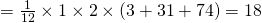Since LHS = RHS, P(1) is true.

Assume P(k) is true for some k,Want to show P(k+1) is true,LHS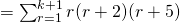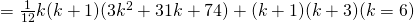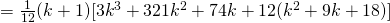= RHS

Since P(1) is true, P(k) is trueP(k+1) is true, hence, by Mathematical Induction, P(n) is true for all(b)
(i)(ii)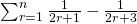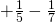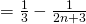(iii)Least n = 499

Not readable? Change text.